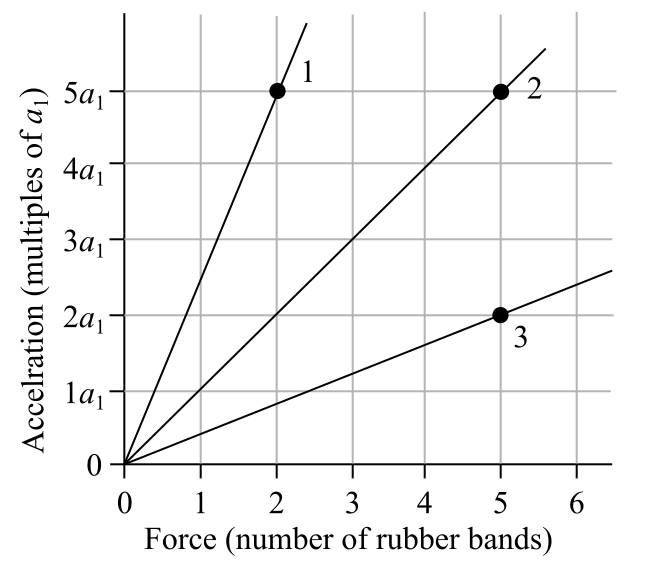# Problem: The figure shows an acceleration-versus-force graph for three objects pulled by rubber bands. The mass of object 2 is 0.30 kg.What is the mass of object 1?

###### FREE Expert Solution

Newton's second law:

$\overline{){\mathbf{\Sigma }}{\mathbf{F}}{\mathbf{=}}{\mathbf{m}}{\mathbf{a}}}$

Solving for a1 from object 2:

F2 = m2a2

5 = (0.30)(5a1)###### Problem Details

The figure shows an acceleration-versus-force graph for three objects pulled by rubber bands. The mass of object 2 is 0.30 kg.

What is the mass of object 1?